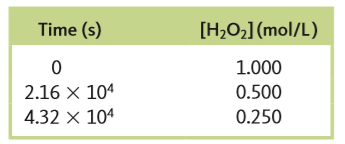# Problem: At 40°C, H2O2(aq) will decompose according to the following reaction:2H2O2 (aq) → 2H2O (l) + O2 (g)The following data were collected for the concentration of H 2O2 at various times.a. Calculate the average rate of decomposition of H 2O2 between 0 and 2.16 x 104 s. Use this rate to calculate the average rate of production of O2(g) over the same time period.

🤓 Based on our data, we think this question is relevant for Professor Tezcan's class at UCSD.

###### FREE Expert Solution

We are asked to calculate the average rate of decomposition of H2O2 and O2 in the given reaction:

2 H2O2 (aq) → 2 H2O (l)   +   O(g)

We’re given the following data:

 Time (s) [H2O2] (mol/L) 0 1.000 2.16 × 104 0.500 4.32 × 104 0.250###### Problem Details

At 40°C, H2O2(aq) will decompose according to the following reaction:

2H2O(aq) → 2H2O (l) + O2 (g)

The following data were collected for the concentration of H 2O2 at various times.a. Calculate the average rate of decomposition of H 2O2 between 0 and 2.16 x 104 s. Use this rate to calculate the average rate of production of O2(g) over the same time period.